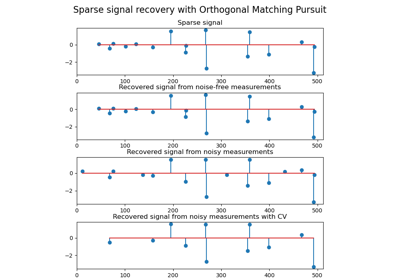# sklearn.datasets.make_sparse_coded_signal¶

sklearn.datasets.make_sparse_coded_signal(n_samples, *, n_components, n_features, n_nonzero_coefs, random_state=None, data_transposed='warn')[source]

Generate a signal as a sparse combination of dictionary elements.

Returns a matrix Y = DX, such that D is of shape (n_features, n_components), X is of shape (n_components, n_samples) and each column of X has exactly n_nonzero_coefs non-zero elements.

Read more in the User Guide.

Parameters:
n_samplesint

Number of samples to generate.

n_componentsint

Number of components in the dictionary.

n_featuresint

Number of features of the dataset to generate.

n_nonzero_coefsint

Number of active (non-zero) coefficients in each sample.

random_stateint, RandomState instance or None, default=None

Determines random number generation for dataset creation. Pass an int for reproducible output across multiple function calls. See Glossary.

data_transposedbool, default=True

By default, Y, D and X are transposed.

New in version 1.1.

Returns:
datandarray of shape (n_features, n_samples) or (n_samples, n_features)

The encoded signal (Y). The shape is (n_samples, n_features) if data_transposed is False, otherwise it’s (n_features, n_samples).

dictionaryndarray of shape (n_features, n_components) or (n_components, n_features)

The dictionary with normalized components (D). The shape is (n_components, n_features) if data_transposed is False, otherwise it’s (n_features, n_components).

codendarray of shape (n_components, n_samples) or (n_samples, n_components)

The sparse code such that each column of this matrix has exactly n_nonzero_coefs non-zero items (X). The shape is (n_samples, n_components) if data_transposed is False, otherwise it’s (n_components, n_samples).

## Examples using sklearn.datasets.make_sparse_coded_signal¶Orthogonal Matching Pursuit

Orthogonal Matching Pursuit